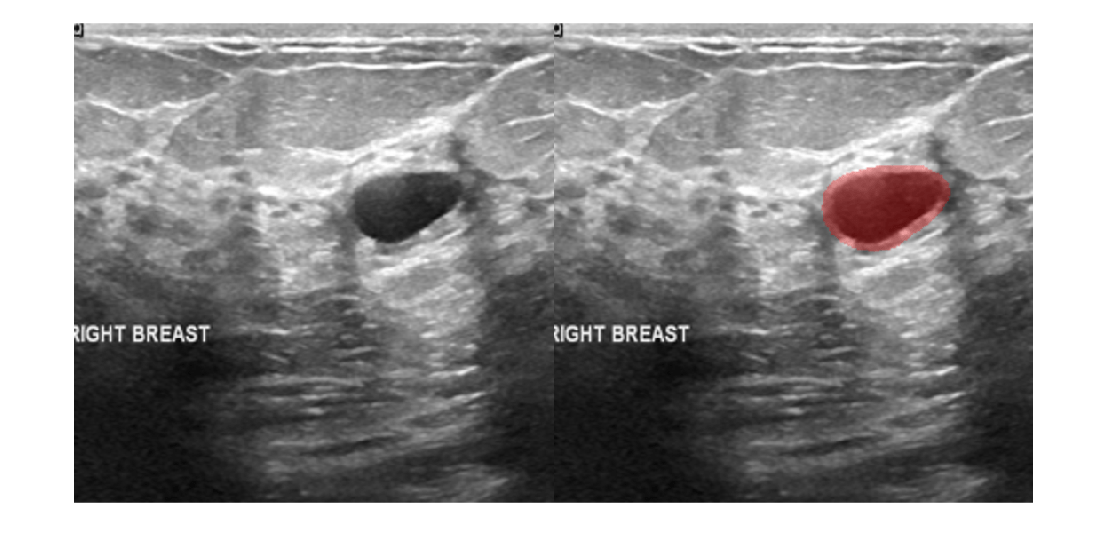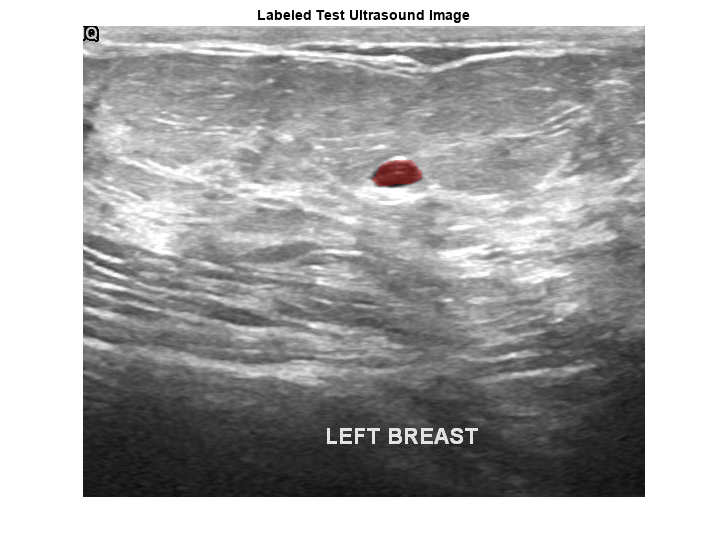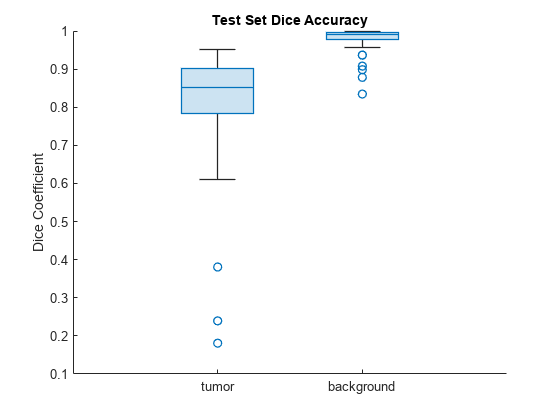# Breast Tumor Segmentation from Ultrasound Using Deep Learning

This example shows how to perform semantic segmentation of breast tumors from 2-D ultrasound images using a deep neural network.

Semantic segmentation involves assigning a class to each pixel in a 2-D image. In this example, you perform breast tumor segmentation using the DeepLab v3+ architecture. A common challenge of medical image segmentation is class imbalance. In segmentation, class imbalance means the size of the region of interest, such as a tumor, is small relative to the image background, resulting in many more pixels in the background class. This example addresses class imbalance by using a custom Tversky loss . The Tversky loss is an asymmetric similarity measure that is a generalization of the Dice index and the Jaccard index.

Create a folder in which to store the pretrained network and image data set. In this example, a folder named `BreastSegmentation` created within the `tempdir` directory has been used as `dataDir`. Download the pretrained DeepLab v3+ network and test image by using the `downloadTrainedNetwork` helper function. The helper function is attached to this example as a supporting file. You can use the pretrained network to run the example without waiting for training to complete.

```dataDir = fullfile(tempdir,"BreastSegmentation"); if ~exist(dataDir,"dir") mkdir(dataDir) end pretrainedNetwork_url = "https://www.mathworks.com/supportfiles/"+ ... "image/data/breastTumorDeepLabV3.tar.gz"; downloadTrainedNetwork(pretrainedNetwork_url,dataDir);```

Unzip the TAR GZ file completely. Load the pretrained network into a variable called `trainedNet`.

```gunzip(fullfile(dataDir,"breastTumorDeepLabV3.tar.gz"),dataDir); untar(fullfile(dataDir,"breastTumorDeepLabV3.tar"),dataDir); exampleDir = fullfile(dataDir,"breastTumorDeepLabV3"); load(fullfile(exampleDir,"breast_seg_deepLabV3.mat"));```

Read the test ultrasound image and resize the image to the input size of the pretrained network.

```imTest = imread(fullfile(exampleDir,"breastUltrasoundImg.png")); imSize = [256 256]; imTest = imresize(imTest,imSize);```

Predict the tumor segmentation mask for the test image.

`segmentedImg = semanticseg(imTest,trainedNet);`

Display the test image and the test image with the predicted tumor label overlay as a montage.

```overlayImg = labeloverlay(imTest,segmentedImg,Transparency=0.7,IncludedLabels="tumor", ... Colormap="hsv"); montage({imTest,overlayImg});```This example uses the Breast Ultrasound Images (BUSI) data set . The BUSI data set contains 2-D ultrasound images stored in the PNG file format. The total size of the data set is 197 MB. The data set contains 133 normal scans, 487 scans with benign tumors, and 210 scans with malignant tumors. This example uses images from the tumor groups only. Each ultrasound image has a corresponding tumor mask image. The tumor mask labels have been reviewed by clinical radiologists .

```zipFile = matlab.internal.examples.downloadSupportFile("image","data/Dataset_BUSI.zip"); filepath = fileparts(zipFile); unzip(zipFile,filepath)```

The `imageDir` folder contains the downloaded and unzipped dataset.

`imageDir = fullfile(filepath,"Dataset_BUSI_with_GT");`

Create an `imageDatastore` object to read and manage the ultrasound image data. Label each image as `normal`, `benign`, or `malignant` according to the name of its folder.

`imds = imageDatastore(imageDir,IncludeSubfolders=true,LabelSource="foldernames");`

Remove files whose names contain `"mask"` to remove label images from the datastore. The image datastore now contains only the grayscale ultrasound images.

`imds = subset(imds,find(~contains(imds.Files,"mask")));`

Create a `pixelLabelDatastore` (Computer Vision Toolbox) object to store the labels.

```classNames = ["tumor","background"]; labelIDs = [1 0]; numClasses = numel(classNames); pxds = pixelLabelDatastore(imageDir,classNames,labelIDs,IncludeSubfolders=true);```

Include only the subset of files whose names contain `"_mask.png`" in the datastore. The pixel label datastore now contains only the tumor mask images.

`pxds = subset(pxds,contains(pxds.Files,"_mask.png"));`

Preview one image with a tumor mask overlay.

```testImage = preview(imds); mask = preview(pxds); B = labeloverlay(testImage,mask,Transparency=0.7,IncludedLabels="tumor", ... Colormap="hsv"); imshow(B) title("Labeled Test Ultrasound Image")```Combine the image datastore and the pixel label datastore to create a `CombinedDatastore` object.

`dsCombined = combine(imds,pxds);`

### Prepare Data for Training

#### Partition Data into Training, Validation, and Test Sets

Split the combined datastore into data sets for training, validation, and testing. Allocate 80% of the data for training, 10% for validation, and the remaining 10% for testing. Determine the indices to include in each set by using the `splitlabels` (Computer Vision Toolbox) function. To exclude images in the `normal` class without tumor images, use the image datastore labels as input and set the `Exclude` name-value argument to `"normal"`.

```idxSet = splitlabels(imds.Labels,[0.8,0.1],"randomized",Exclude="normal"); dsTrain = subset(dsCombined,idxSet{1}); dsVal = subset(dsCombined,idxSet{2}); dsTest = subset(dsCombined,idxSet{3});```

#### Augment Training and Validation Data

Augment the training and validation data by using the `transform` function with custom preprocessing operations specified by the `transformBreastTumorImageAndLabels` helper function. The helper function is attached to the example as a supporting file. The `transformBreastTumorImageAndLabels` function performs these operations:

1. Convert the ultrasound images from RGB to grayscale.

2. Augment the intensity of the grayscale images by using the `jitterIntensity` (Medical Imaging Toolbox) function.

3. Resize the images to 256-by-256 pixels.

```tdsTrain = transform(dsTrain,@transformBreastTumorImageAndLabels,IncludeInfo=true); tdsVal = transform(dsVal,@transformBreastTumorImageAndLabels,IncludeInfo=true);```

### Define Network Architecture

This example uses the DeepLab v3+ network. DeepLab v3+ consists of a series of convolution layers with a skip connection, one maxpool layer, and one averagepool layer. The network also has a batch normalization layer before each ReLU layer.

Create a DeepLab v3+ network based on ResNet-50 by using the using `deeplabv3plusLayers` (Computer Vision Toolbox) function. Setting the base network as ResNet-50 requires the Deep Learning Toolbox™ Model for ResNet-50 Network support package. If this support package is not installed, then the function provides a download link.

Define the input size of the network as 256-by-256-by-3. Specify the number of classes as two for `background` and `tumor`.

```imageSize = [256 256 3]; lgraph = deeplabv3plusLayers(imageSize,numClasses,"resnet50");```

Because the preprocessed ultrasound images are grayscale, replace the original input layer with a 256-by-256 input layer.

```newInputLayer = imageInputLayer(imageSize(1:2),Name="newInputLayer"); lgraph = replaceLayer(lgraph,lgraph.Layers(1).Name,newInputLayer);```

Replace the first 2-D convolution layer with a new 2-D convolution layer to match the size of the new input layer.

```newConvLayer = convolution2dLayer([7 7],64,Stride=2,Padding=[3 3 3 3],Name="newConv1"); lgraph = replaceLayer(lgraph,lgraph.Layers(2).Name,newConvLayer);```

To better segment smaller tumor regions and reduce the influence of larger background regions, use a custom Tversky pixel classification layer. For more details about using a custom Tversky layer, see Define Custom Pixel Classification Layer with Tversky Loss. Replace the pixel classification layer with the Tversky pixel classification layer. The `alpha` and `beta` weighting factors control the contribution of false positives and false negatives, respectively, to the loss function. The `alpha` and `beta` values used in this example were selected using trial and error for the target data set. Generally, specifying the `beta` value greater than the `alpha` value is useful for training images with small objects and large background regions.

```alpha = 0.01; beta = 0.99; pxLayer = tverskyPixelClassificationLayer("tverskyLoss",alpha,beta); lgraph = replaceLayer(lgraph,"classification",pxLayer);```

Alternatively, you can modify the DeepLab v3+ network by using the Deep Network Designer from Deep Learning Toolbox.

Use the Analyze tool in the Deep Network Designer to analyze the DeepLab v3+ network.

`deepNetworkDesigner(lgraph)`

### Specify Training Options

Train the network using the `adam` optimization solver. Specify the hyperparameter settings using the `trainingOptions` function. Set the learning rate to `1e-3` over the span of training. You can experiment with the mini-batch size based on your GPU memory. Batch normalization layers are less effective for smaller values of the mini-batch size. Tune the initial learning rate based on the mini-batch size.

```options = trainingOptions("adam", ... ExecutionEnvironment="gpu", ... InitialLearnRate=1e-3, ... ValidationData=tdsVal, ... MaxEpochs=300, ... MiniBatchSize=16, ... VerboseFrequency=20, ... Plots="training-progress");```

### Train Network

To train the network, set the `doTraining` variable to `true`. Train the model using the `trainNetwork` function.

Train on a GPU if one is available. Using a GPU requires Parallel Computing Toolbox™ and a CUDA® enabled NVIDIA® GPU. For more information, see GPU Computing Requirements (Parallel Computing Toolbox). Training takes about four hours on a single-GPU system with an NVIDIA™ Titan Xp GPU and can take longer depending on your GPU hardware.

```doTraining =false; if doTraining [trainedNet,info] = trainNetwork(tdsTrain,lgraph,options); modelDateTime = string(datetime("now",Format="yyyy-MM-dd-HH-mm-ss")); save("breastTumorDeepLabv3-"+modelDateTime+".mat","trainedNet"); end```

### Predict Using New Data

#### Preprocess Test Data

Prepare the test data by using the `transform` function with custom preprocessing operations specified by the `transformBreastTumorImageResize` helper function. This helper function is attached to the example as a supporting file. The `transformBreastTumorImageResize` function converts images from RGB to grayscale and resizes the images to 256-by-256 pixels.

`dsTest = transform(dsTest,@transformBreastTumorImageResize,IncludeInfo=true);`

#### Segment Test Data

Use the trained network for semantic segmentation of the test data set.

`pxdsResults = semanticseg(dsTest,trainedNet,Verbose=true);`
```Running semantic segmentation network ------------------------------------- * Processed 65 images. ```

#### Evaluate Segmentation Accuracy

Evaluate the network-predicted segmentation results against the ground truth pixel label tumor masks.

`metrics = evaluateSemanticSegmentation(pxdsResults,dsTest,Verbose=true);`
```Evaluating semantic segmentation results ---------------------------------------- * Selected metrics: global accuracy, class accuracy, IoU, weighted IoU, BF score. * Processed 65 images. * Finalizing... Done. * Data set metrics: GlobalAccuracy MeanAccuracy MeanIoU WeightedIoU MeanBFScore ______________ ____________ _______ ___________ ___________ 0.97588 0.96236 0.86931 0.9565 0.57667 ```

Measure the segmentation accuracy using the `evaluateBreastTumorDiceAccuracy` helper function. This helper function computes the Dice index between the predicted and ground truth segmentations using the `dice` (Image Processing Toolbox) function. The helper function is attached to the example as a supporting file.

`[diceTumor,diceBackground,numTestImgs] = evaluateBreastTumorDiceAccuracy(pxdsResults,dsTest);`

Calculate the average Dice index across the set of test images.

```disp("Average Dice score of background across "+num2str(numTestImgs)+ ... " test images = "+num2str(mean(diceBackground)))```
```Average Dice score of background across 65 test images = 0.98581 ```
```disp("Average Dice score of tumor across "+num2str(numTestImgs)+ ... " test images = "+num2str(mean(diceTumor)))```
```Average Dice score of tumor across 65 test images = 0.78588 ```
```disp("Median Dice score of tumor across "+num2str(numTestImgs)+ ... " test images = "+num2str(median(diceTumor)))```
```Median Dice score of tumor across 65 test images = 0.85888 ```

Visualize statistics about the Dice scores as a box chart. The middle blue line in the plot shows the median Dice index. The upper and lower bounds of the blue box indicate the 25th and 75th percentiles, respectively. Black whiskers extend to the most extreme data points that are not outliers.

```figure boxchart([diceTumor diceBackground]) title("Test Set Dice Accuracy") xticklabels(classNames) ylabel("Dice Coefficient")```### References

 Salehi, Seyed Sadegh Mohseni, Deniz Erdogmus, and Ali Gholipour. “Tversky Loss Function for Image Segmentation Using 3D Fully Convolutional Deep Networks.” In Machine Learning in Medical Imaging, edited by Qian Wang, Yinghuan Shi, Heung-Il Suk, and Kenji Suzuki, 10541:379–87. Cham: Springer International Publishing, 2017. https://doi.org/10.1007/978-3-319-67389-9_44.

 Al-Dhabyani, Walid, Mohammed Gomaa, Hussien Khaled, and Aly Fahmy. “Dataset of Breast Ultrasound Images.” Data in Brief 28 (February 2020): 104863. https://doi.org/10.1016/j.dib.2019.104863.